### Problem Statement

You are given a square black-and-white image divided into two parts, each part filled with its own texture. Your task is to separate the parts and return the corresponding marking of the image.

### Implementation

You will implement one method recognize. image is a square image SxS pixels (S can be determined as number of elements in image), character j of image[i] representing the color of the pixel in row i and column j, '0' for black and '1' for white. You must return a marking of the image in the same format, '0' for a pixel that belongs to one texture, and '1' for a pixel that belongs to the other texture. It doesn't matter which texture you mark with '0's, the marking which results in higher percentage of correctly marked pixels is used.

### Image generation

To generate a texture, the number of axes is chosen between 2 and 4, inclusive, each being vertical (dx=0, dy=1), horizontal (dx=1, dy=0) or diagonal (dx=dy=1 or dx=-dy=1). Several axes of the set can have the same direction. For each axis, its "weight" is chosen between 1 and 5.

The neighborhood of a pixel (x,y) for a chosen set of axes is generated as follows:
```    pixel (x,y) is added to the neighborhood
for each axis
for k = 1 .. weight
pixels ((x+dx*k+S)%S,(y+dy*k+S)%S) and ((x-dx*k+S)%S,(y-dy*k+S)%S) are added to the neighborhood
```
Note that the neighborhood of a pixel is a multiset and can contain each pixel more than once.

The initial state of the texture is generated randomly by assigning each pixel color '0' or '1'.

The procedure of adjusting a pixel's color is as follows:
```    if the number of '1's in its neighborhood is strictly greater than the number of '0's
set the color of the pixel to '1'
else
set the color of the pixel to '0'
```
The image is then modified iteratively:
```    for k = 1 .. 2*S*S
pick random pixel
for i = 1 .. S
for j = 1 .. S
pick pixel (i,j)
```
Finally, the image is inverted with a probability of 1/3.

To generate an image with two textures, two images are generated independently, as above. We then use a stochastic flood fill algorithm to determine which pixels of the final image are colored according to which texture. Four (unique) pixels out of an SxS pixel image are chosen randomly. Two of these are colored black, and two are colored blue. We then perform the following to color the rest of the image, where neighbor means adjacent in one of the four cardinal directions:
```    while(there is an uncolored pixel)
pick a colored pixel (i,j) with an uncolored neighbor (ii,jj)
color the (ii,jj) with the color of (i,j)
```
If this procedure produces more than two colored regions (a colored region is a connected component of all the same color), it is repeated from scratch.

Finally, the black pixels are replaced with the pixel values from one texture, and the blue pixels are replaced with the pixel values from the second texture.

### Scoring

Your score for an individual test case will be the number of pixels you marked correctly multiplied by 100/(S*S). Invalid return (wrong number of elements in return, or characters other than '0' or '1') will result in 0 score for that test case.

Your overall score will be the sum of your individual scores.

### Tools

A visualization tool is provided at http://www.topcoder.com/contest/problem/Textures/vis.html

### Definition

 Class: Textures Method: recognize Parameters: String[] Returns: String[] Method signature: String[] recognize(String[] image) (be sure your method is public)

### Notes

-The time limit is 10 seconds and the memory limit is 1024M.
-There are 10 example test cases and 500 full submission test cases.
-For more details on the image generation, see the visualizer source.

### Constraints

-S will be between 100 and 300, inclusive, and will be divisible by 10.

### Examples

0)

 `"1"`
```Returns:
"Seed = 1
S = 260
Texture:<br/>
dx = 1, dy = 0, w = 1<br/>
dx = 1, dy = 0, w = 2<br/>
Texture:<br/>
dx = 0, dy = 1, w = 5<br/>
dx = 1, dy = -1, w = 2<br/>
"```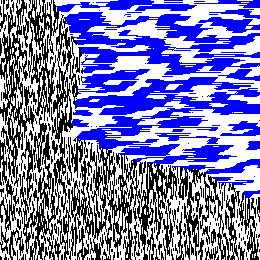1)

 `"2"`
```Returns:
"Seed = 2
S = 160
Texture:<br/>
dx = 0, dy = 1, w = 1<br/>
dx = 1, dy = 1, w = 5<br/>
Texture:<br/>
dx = 1, dy = -1, w = 2<br/>
dx = 0, dy = 1, w = 4<br/>
dx = 1, dy = -1, w = 4<br/>
dx = 1, dy = 0, w = 4<br/>
"```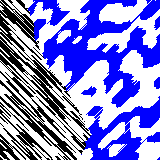2)

 `"3"`
```Returns:
"Seed = 3
S = 290
Texture:<br/>
dx = 0, dy = 1, w = 4<br/>
dx = 1, dy = 1, w = 5<br/>
Texture:<br/>
dx = 1, dy = 1, w = 4<br/>
dx = 1, dy = -1, w = 5<br/>
dx = 0, dy = 1, w = 3<br/>
"```3)

 `"4"`
```Returns:
"Seed = 4
S = 100
Texture:<br/>
dx = 0, dy = 1, w = 3<br/>
dx = 1, dy = 1, w = 1<br/>
Texture:<br/>
dx = 1, dy = -1, w = 4<br/>
dx = 1, dy = -1, w = 1<br/>
"```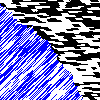4)

 `"5"`
```Returns:
"Seed = 5
S = 270
Texture:<br/>
dx = 0, dy = 1, w = 3<br/>
dx = 1, dy = -1, w = 5<br/>
Texture:<br/>
dx = 1, dy = -1, w = 1<br/>
dx = 1, dy = 1, w = 2<br/>
dx = 1, dy = 1, w = 3<br/>
dx = 0, dy = 1, w = 5<br/>
"```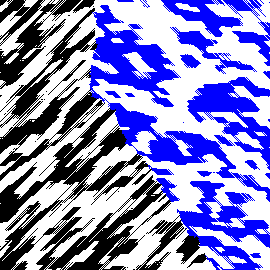5)

 `"6"`
```Returns:
"Seed = 6
S = 130
Texture:<br/>
dx = 1, dy = 1, w = 1<br/>
dx = 1, dy = 0, w = 1<br/>
Texture:<br/>
dx = 1, dy = 0, w = 1<br/>
dx = 0, dy = 1, w = 1<br/>
dx = 0, dy = 1, w = 5<br/>
"```6)

 `"7"`
```Returns:
"Seed = 7
S = 280
Texture:<br/>
dx = 1, dy = 0, w = 2<br/>
dx = 1, dy = 1, w = 5<br/>
dx = 1, dy = 1, w = 2<br/>
dx = 0, dy = 1, w = 1<br/>
Texture:<br/>
dx = 0, dy = 1, w = 3<br/>
dx = 0, dy = 1, w = 2<br/>
"```7)

 `"8"`
```Returns:
"Seed = 8
S = 140
Texture:<br/>
dx = 1, dy = 1, w = 4<br/>
dx = 0, dy = 1, w = 4<br/>
Texture:<br/>
dx = 1, dy = 0, w = 2<br/>
dx = 1, dy = 1, w = 1<br/>
dx = 1, dy = 1, w = 4<br/>
"```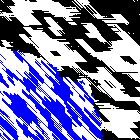8)

 `"9"`
```Returns:
"Seed = 9
S = 200
Texture:<br/>
dx = 1, dy = -1, w = 3<br/>
dx = 1, dy = -1, w = 4<br/>
Texture:<br/>
dx = 0, dy = 1, w = 3<br/>
dx = 0, dy = 1, w = 2<br/>
"```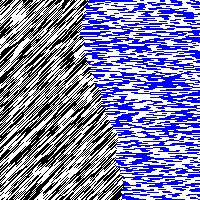9)

 `"10"`
```Returns:
"Seed = 10
S = 140
Texture:<br/>
dx = 0, dy = 1, w = 2<br/>
dx = 1, dy = -1, w = 4<br/>
dx = 1, dy = -1, w = 4<br/>
Texture:<br/>
dx = 1, dy = 0, w = 2<br/>
dx = 1, dy = 1, w = 2<br/>
dx = 0, dy = 1, w = 4<br/>
dx = 1, dy = 1, w = 2<br/>
"```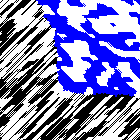#### Problem url:

http://www.topcoder.com/stat?c=problem_statement&pm=8737

#### Problem stats url:

http://www.topcoder.com/tc?module=ProblemDetail&rd=12203&pm=8737

Unknown

Math